﻿ CreateFrom Method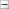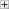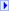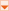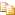Creates an instance of AsymmetricKeyAlgorithm from the specified asymmetric algorithm object.

Namespace:  Rebex.Security.Cryptography
Assembly:  Rebex.Common (in Rebex.Common.dll)

#Syntax

Visual Basic
```Public Shared Function CreateFrom ( _
algorithm As AsymmetricAlgorithm, _
ownsAlgorithm As Boolean _
) As AsymmetricKeyAlgorithm```
C#
```public static AsymmetricKeyAlgorithm CreateFrom(
AsymmetricAlgorithm algorithm,
bool ownsAlgorithm
)```

#### Parameters

algorithm
Type: System.Security.Cryptography..::..AsymmetricAlgorithm
Asymmetric algorithm object.
ownsAlgorithm
Type: System..::..Boolean
True if the created object owns the asymmetric algorithm object.

#### Return Value

An instance of AsymmetricKeyAlgorithm.

#Version Information

#### .NET Compact Framework

Supported in: 3.9, 3.5